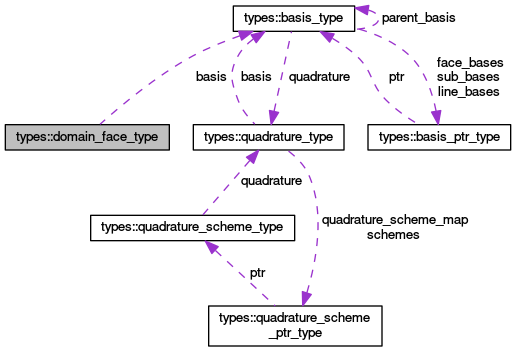OpenCMISS-Iron Internal API Documentation
types::domain_face_type Type Reference

Contains the information for a face in a domain. More...

Collaboration diagram for types::domain_face_type:[legend]

## Public Attributes

integer(intg) number
The face number in the domain. More...

integer(intg) xi_direction1
The first xi direction of the face. More...

integer(intg) xi_direction2
The second xi direction of the face. More...

type(basis_type), pointer basis
A pointer to the basis function for the face. More...

integer(intg), dimension(:), allocatable nodes_in_face
NODES_IN_FACE(nn). The local node number in the domain of the nn'th local node in the face. Old CMISS name NPNF(nn,nbf). More...

integer(intg), dimension(:,:,:), allocatable derivatives_in_face
DERIVATIVES_IN_FACE(i,local_derivative_idx,local_node_idx). When i=1 DERIVATIVES_IN_FACE will give the global derivative number of the local derivative_idx'th local derivative for the local_node_idx'th local node in the face, When i=2 DERIVATIVES_IN_FACE will give the global derivative version number of the local derivative_idx'th local derivative for the local_node_idx'th local node in the face. More...

logical boundary_face
Is .TRUE. if the face is on the boundary of the mesh for the domain, .FALSE. if not. More...

integer(intg) element_number
The element number of the element on which the face is on. More...

## Detailed Description

Contains the information for a face in a domain.

Definition at line 644 of file types.f90.

## Member Data Documentation

 type(basis_type), pointer types::domain_face_type::basis

A pointer to the basis function for the face.

Definition at line 648 of file types.f90.

 logical types::domain_face_type::boundary_face

Is .TRUE. if the face is on the boundary of the mesh for the domain, .FALSE. if not.

Definition at line 651 of file types.f90.

 integer(intg), dimension(:,:,:), allocatable types::domain_face_type::derivatives_in_face

DERIVATIVES_IN_FACE(i,local_derivative_idx,local_node_idx). When i=1 DERIVATIVES_IN_FACE will give the global derivative number of the local derivative_idx'th local derivative for the local_node_idx'th local node in the face, When i=2 DERIVATIVES_IN_FACE will give the global derivative version number of the local derivative_idx'th local derivative for the local_node_idx'th local node in the face.

Definition at line 650 of file types.f90.

 integer(intg) types::domain_face_type::element_number

The element number of the element on which the face is on.

Definition at line 652 of file types.f90.

 integer(intg), dimension(:), allocatable types::domain_face_type::nodes_in_face

NODES_IN_FACE(nn). The local node number in the domain of the nn'th local node in the face. Old CMISS name NPNF(nn,nbf).

Definition at line 649 of file types.f90.

 integer(intg) types::domain_face_type::number

The face number in the domain.

Definition at line 645 of file types.f90.

 integer(intg) types::domain_face_type::xi_direction1

The first xi direction of the face.

Todo:
move this to the decomposition face type

Definition at line 646 of file types.f90.

 integer(intg) types::domain_face_type::xi_direction2

The second xi direction of the face.

Todo:
move this to the decomposition face type

Definition at line 647 of file types.f90.